## Monday, September 26, 2016

### Bayes's Theorem is not optional

Abstract: I present a probability puzzle, the Rain in Seattle Problem, and use it to explain differences between the Bayesian and frequentist interpretations of probability, and between Bayesian and frequentist statistical methods.  Since I am trying to clear up confusion, I try to describe the alternatives without commenting on their pros and cons.

### Introduction

Conversations about Bayesian statistics sometimes get bogged down in confusion about two separate questions:

1) The Bayesian interpretation of probability, as opposed to the frequentist interpretation.

2) The Bayesian approach to statistical inference, as opposed to frequentist approach.

The first is a philosophical position about what probability means; the second is more like a practical recommendation about how to make inferences from data.  They are almost entirely separate questions; for example, you might prefer the Bayesian interpretation of probability by philosophical criteria, and then use frequentist statistics because of practical requirements; or the other way around.

Under the frequentist interpretation of probability, we can only talk about the probability of an event if we can model it as a subset of a sample space.  For example, we can talk about the probability of drawing a straight in poker because a straight is a well-defined subset of the sample space that contains all poker hands.  But by this interpretation, we could not assign a probability to the proposition that Hillary Clinton will win the election, unless we could model this event as a subset of all elections, somehow.

Under the Bayesian interpretation, a probability represents a degree of belief, so it is permissible to assign probabilities to events even if they are unique.  It is also permissible to use probability to represent uncertainty about non-random events.  For example, if you are uncertain about whether there is life on Mars, you could assign a probability to that proposition under the Bayesian interpretation.  Under the frequentist interpretation, there either is life on Mars or not; it is not a random event, so we can't assign a probability to it.

(I avoid saying things like "a Bayesian believes this" or "a Frequentist believes that".  These are philosophical positions, and we can discuss their consequences regardless of who believes what.)

In problems where the frequentist interpretation of probability applies, the Bayesian and frequentist interpretations yield the same answers.  The difference is that for some problems we get an answer under Bayesianism and no answer under frequentism.

Now, before I get into Bayesian and frequentist inference, let's look at an example.

### The Rain in Seattle problem

Suppose you are interviewing for a data science job and you are asked this question (from glassdoor.com):
You're about to get on a plane to Seattle. You want to know if you should bring an umbrella. You call 3 random friends of yours who live there and ask each independently if it's raining. Each of your friends has a 2/3 chance of telling you the truth and a 1/3 chance of messing with you by lying. All 3 friends tell you that "Yes" it is raining. What is the probability that it's actually raining in Seattle?
Take a minute to think about it before you go on.  Then take a look at the responses on glassdoor.com. The top response, which uses Bayes's Theorem, is correct.  I'll explain the correct solution first; then I want to comment on some of the other responses.

The question asks you to compute the probability of rain conditioned on three yesses, which I'll write P(rain|YYY).

Now, here's an important point: you can't give a meaningful answer to this question unless you know P(rain), the probability of rain unconditioned on what your friends say.   To see why, consider two extreme cases:

1. If P(rain) is 1, it always rains in Seattle.  If your friends all tell you it's raining, you know that they are telling the truth, and that P(rain|YYY) is 1.

2. If P(rain) is 0, it never rains in Seattle, so you know your friends are lying and P(rain|YYY) = 0.

For values of P(rain) between 0 and 1, the answer could be any value between 0 and 1.  So if you see any response to this question that does not take into account P(rain), you can be sure that it is wrong (or coincidentally right based on an invalid argument).

But if we are given the base rate, we can solve the problem easily using Bayes's Rule,   According to the Western Regional Climate Center, from 1965-99 there was measurable rain in Seattle during 822 hours per year, which is about 10% of the time.

A base rate of 10% corresponds to prior odds of 1:9.  Each friend is twice as likely to tell the truth as to lie, so each friend contributes evidence in favor of rain with a likelihood ratio, or Bayes factor, of 2.  Multiplying the prior odds by the likelihood ratios yields posterior odds 8:9, which corresponds to probability 8/17, or 0.47.

And that is the unique correct answer to the question (provided that you accept the modeling assumptions).  More generally, if P(rain) = p, the conditional probability P(rain|YYY) is

Probability(8 Odds(p))

assuming that Odds() converts probabilities to odds and Probability() does the opposite.

Several of the responses on glassdoor.com provide what they call a frequentist or non-Bayes perspective:
Answer from a frequentist perspective:  Suppose there was one person. P(Y|rain) is twice (2/3 / 1/3) as likely as P(Y|no rain), so the P(rain) is 2/3.  If instead n people all say YES, then they are either all telling the truth, or all lying. The outcome that they are all telling the truth is (2/3)^n / (1/3)^n = 2^n as likely as the outcome that they are not. Thus P(YYY | rain) = 2^n / (2^n + 1) = 8/9 for n=3.  Notice that this corresponds exactly to the Bayesian answer when prior(raining) = 1/2.
And here's another:
I thought about this a little differently from a non-Bayes perspective.  It's raining if any ONE of the friends is telling the truth, because if they are telling the truth then it is raining. If all of them are lying, then it isn't raining because they told you that it was raining.  So what you want is the probability that any one person is telling the truth.  Which is simply 1-Pr(all lie) = 26/27.  Anyone let me know if I'm wrong here!
These are not actually frequentist responses.  For this problem, we get the same answer under Bayesianism and frequentism because:

1) Everything in this problem can be well-modeled by random processes.  There is a well-defined long-run probability of rain in Seattle, and we can model the friends' responses as independent random variables (at least according to the statement of the problem).

AND

2) There is nothing especially Bayesian about Bayes's Theorem!  Bayes's Theorem is an uncontroversial law of probability that is true under any interpretation of probability, and can be used for any kind of statistical inference.

The "non-Bayes" responses are not actually other perspectives; they are just incorrect.  Under frequentism, we would either accept the solution based on Bayes's Theorem or, under a strict interpretation, we might say that it is either raining in Seattle or not, and refuse to assign a probability.

### But what about frequentist inference?

Statistical inference is the process of inferring the properties of a population based on a sample.  For example, if you want to know the fraction of U.S. voters who intend to vote for Donald Trump, you could poll a sample of the population.  Then,

1) Using frequentist inference, you could compute an estimate of the fraction of the population that intends to vote for Trump (call it x), you could compute a confidence interval for the estimate, and you could compute a p-value based on a null-hypothesis like "x is 50%".  But if anyone asked "what's the probability that x is greater than 50%", you would not be able to answer that question.

2) Using Bayesian inference, you would start with some prior belief about x, use the polling data to update your belief, and produce a posterior distribution for x, which represents all possible values and their probabilities.  You could use the posterior distribution to compute estimates and intervals similar to the results of frequentist inference.  But if someone asked "what's the probability that x is greater than 50%", you could compute the answer easily.

So, how does this apply to the Rain in Seattle Problem?  It doesn't, because the Rain in Seattle problem has nothing to do with statistical inference.  It is a question about probability, not statistics. It has one correct answer under any interpretation of probability, regardless of your preferences for statistical inference.

### Summary

1) Conversations about Bayesian methods will be improved if we distinguish two almost unrelated questions: the meaning of probability and the choice of inferential methods.

2) You don't have to be a Bayesian to use Bayes's Theorem.  Most probability problems, including the Rain in Seattle problem, have a single solution considered correct under any interpretation of probability and statistics.

UPDATE October 13, 2015:  A few people asked me how to solve this problem using Bayes's Theorem (based on probabilities) rather than Bayes's Rule (based on odds).  To apply Bayes's Theorem, you might find it helpful to use my "Bayesian update worksheet".  Here's one that shows how to solve this problem:

## Friday, September 16, 2016

### Blow it up and start again

The president of Olin College, Rick Miller, spoke recently at the Business Innovation Factory.  Here's the most-tweeted quote from the talk: "The only way to change education is to blow it up and start again."

I agree, and I saw an example recently that helps make the point.  The American Statistical Association recently published this Statement on p-Values.  Here's how it starts:
In February 2014, George Cobb, Professor Emeritus of Mathematics
and Statistics at Mount Holyoke College, posed these
questions to an ASA discussion forum:

Q: Why do so many colleges and grad schools teach p = 0.05?
A: Because that’s still what the scientific community and journal
editors use.
Q: Why do so many people still use p = 0.05?
A: Because that’s what they were taught in college or grad school.

Cobb’s concern was a long-worrisome circularity in the sociology
of science based on the use of bright lines such as p < 0.05:
“We teach it because it’s what we do; we do it because it’s what
we teach.”
This "worrisome circularity" is a concrete example of why gradual change is so hard, and why sometimes the only solution is to blow it up and start again.  That idea is scary to a lot of people, but it doesn't have to be.  I have an example that might help, the statistics curriculum at Olin.

### Statistics at Olin

First I'll explain what worked, then we'll look at what could have gone wrong.

In 2010, I proposed a new class called Computational Probability and Statistics, as a substitute for a very conventional statistics class that was offered at the time.  My class was based on a library I developed while I was on sabbatical at Google, which is now the thinkstats module in ThinkX.

While teaching the class, I wrote Think Stats, which was published by O'Reilly Media in 2011. After a few semesters, I developed another course called Computational Bayesian Statistics, and wrote Think Bayes, which was published in 2013.

In 2014 I expanded CompProbStat from 2 credits to 4 and renamed it Data Science.  I recruited external collaborators to provide data and motivating questions for student projects, and several other professors sat in and helped guide student projects.  In 2016 one of those professors took over and taught his version of the class, adding his expertise in machine learning.

At the same time, two of my colleagues were developing their own statistics classes, focused on applications in diverse areas of engineering and science.   None of these classes look much like the conventional statistics material, and they are much better for it.

In six years, we developed five new classes, published two books, got six additional professors involved in teaching data science and statistics, and, most importantly, we developed a curriculum that serves the goals and needs of our students.

### How did that happen?

This project would have been impossible at almost any other college.

At most colleges and universities, a professor of computer science (like me) who proposes a new statistics class will not get far, because of two fundamental and unquestioned assumptions of undergraduate education: (1) you need a Ph.D. in a topic before you can teach an introductory course, and (2) if you do research in a field, that makes you better at teaching it to undergraduates.  Note: neither of these is true.

And the content of my courses would have been scrutinized by a committee with a checklist.  To teach something new, you have to stop teaching something old, and it is nearly impossible to get permission to stop teaching anything.  Every topic, no matter how obsolete, is defended by zealots with no respect for evidence or reason.   Fun example: here are Time magazine's Five Reasons Kids Should Still Learn Cursive Writing.  Note: none of them are good.

Every field has its obstructionists, but statistics has its own special kind: the anti-Bayesians.  I can only imagine the howls if I proposed teaching Bayesian statistics to undergraduates.  When I suggested teaching it before classical statistics, I would have been thrown out a window.  And when I proposed to teach it instead of classical statistics, I would have been dragged through the streets.

At Olin, fixing engineering education is our mission.  When someone proposes an experiment, we ask the right questions: Does it contribute to our mission by improving undergraduate education at Olin and other institutions?  Is it a reasonable risk?  And do we have the resources to do it?  If the answers are yes, we do it.  Note: if it's an unreasonable risk and we don't have the resources, sometimes we do it anyway.

The second reason my project would be impossible at most schools is that statistics is owned by the math or statistics department, and even though the faculty don't like teaching classes for non-majors, they get credit for providing "service classes" (a term I would like to ban), so they have an incentive to protect their territory.

And just as the math department would fight to keep me out, the computer science department would fight to keep me in.  If the CS department owns my "faculty line" (another term I would like to ban), they want me to teach CS classes.

At Olin, we have no departments.  We don't have to do this kind of bookkeeping, and that leaves us free to think about the students (remember them?) and design a curriculum that serves their needs.

The third reason my project wouldn't happen anywhere else is that I wouldn't do it anywhere else.  At most universities, there is no incentive to develop new classes; in fact, there is a strong disincentive.  If you try something new, you make enemies, because the new is an insult to the old.  If it doesn't work, you get punished, and even if it works, you get no reward.

The one factor that drives hiring and firing is research.  Even at liberal arts colleges that value good teaching, there is no expectation for innovation.  If you do a decent job of teaching the same two or three classes over and over, that's good enough.  At Olin, we are encouraged to take risks, supported while we work out the bugs, and rewarded for the effort.

Also, at most universities, there is no incentive to write textbooks.  They don't count as research and they don't count as teaching; the time you spend on a textbook is just time you didn't spend on research.  At Olin, we use broad categories to evaluate faculty work, and a successful textbook is valued because it benefits students (at Olin and other institutions) and contributes to our mission to change engineering education.

### So blow it up

You don't get a lot of opportunities to blow it up and start again, but when you do, a lot of good things can happen.  It's not as scary as it sounds.

Also, there is nothing special about p = 0.05.

## Wednesday, September 14, 2016

### It's a small world, scale-free network after all

Real social networks generally have the properties of small world graphs (high clustering and low path lengths) and the characteristics of scale free networks (a heavy-tailed degree distribution).

The Watts-Strogatz (WS) network model has small world characteristics, but the degree distribution is roughly normal, very different from observed distributions.

The Barabasi-Albert (BA) model has low path lengths and a heavy-tailed degree distribution, but

1. It has low clustering, and
2. The degree distribution does not fit observed data well.

The Holmes-Kim (HK) model generates graphs with higher clustering, although still not as high as observed values. And the degree distribution is heavy tailed, but it still doesn't fit observed distributions well.

I propose a new model that generates graphs with

1. Low path lenths,
2. Clustering coefficients similar to the HK model (but still lower than observed values), and
3. A degree distribution that fits observed data well.

I test the models with a relatively small dataset from SNAP.

The proposed model is based on a "friend of a friend" growth mechanism that is a plausible description of the way social networks actually grow. The implementation is simple, comparable to BA and HK in both lines of code and run time.

All the details are in this Jupyter notebook, but I summarize the primary results here.

### Comparing the models

The Facebook dataset from SNAP contains 4039 nodes and 88234 edges.  The mean path length is 3.7 and the clustering coefficient is 0.6.

A WS model with the same number of nodes and edges, and with probability of rewiring, p=0.05, has mean path length 3.2 and clustering 0.62, so it clearly has the small world properties.  But the distribution of degree does not match the data at all: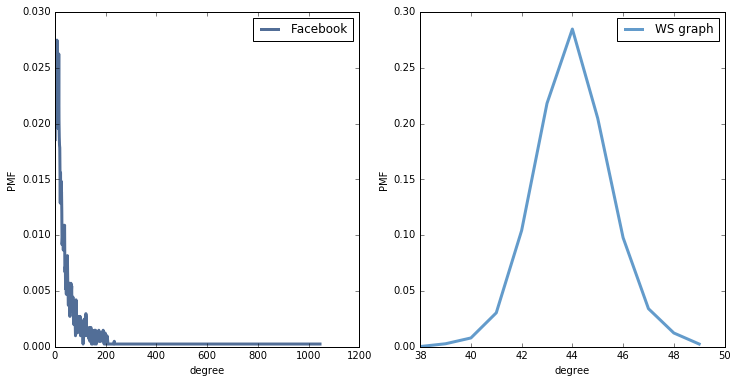A BA model with the same number of nodes and edges has very short paths (2.5), but very low clustering (0.04).  The degree distribution is a better match for the data: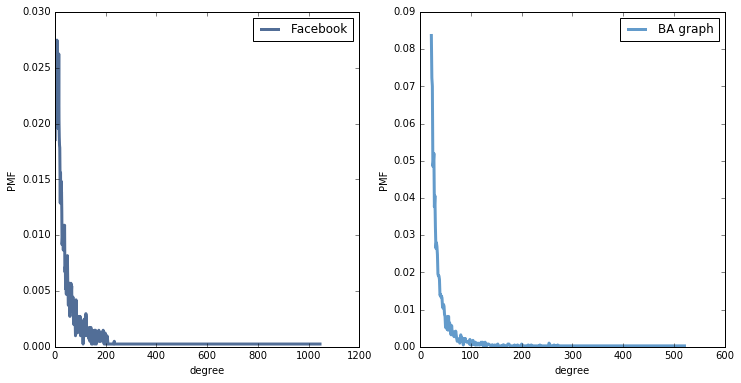If we plot CDFs on a log-log scale, the BA model matches the tail of the distribution reasonably well, but the WS model is hopeless.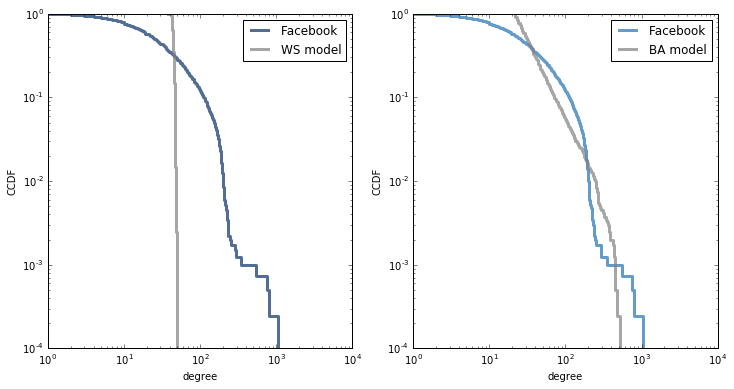But if we plot CDFs on a log-x scale, we see that the BA model does not match the rest of the distribution: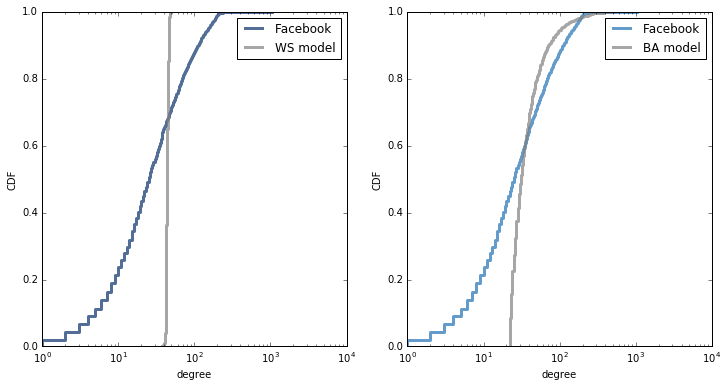The HK model also has short path lengths (2.8), and the clustering is much better (0.23), but still not as high as in the data (0.6).  The degree distribution is pretty much the same as in the BA model.

The FOF model

The generative model I propose is called FOF for "friends of friends". It is similar to both BA and HK, but it yields a degree distribution that matches observed data better.

It starts with a complete graph with m nodes, so initially all nodes have degree m. Each time we generate a node we:

1. Select a random target uniformly from existing nodes.
2. Iterate through the friends of the target. For each one, with probability p, we form a triangle that includes the source, friend, and a random friend of friend.
3. Finally, we connect the source and target.

Because we choose friends of the target, this process has preferential attachment, but it does not yield a power law tail. Rather, the degree distribution is approximately lognormal with median degree m.
Because this process forms triangles, it yields a moderately high clustering coefficient.

A FOF graph with the same number of nodes and edges as the Facebook data has low path length (3.0) and moderate clustering (0.24, which is more than BA, comparable to HK, but still less than the observed value, 0.6).

The degree distribution is a reasonable match for the tail of the observed distribution: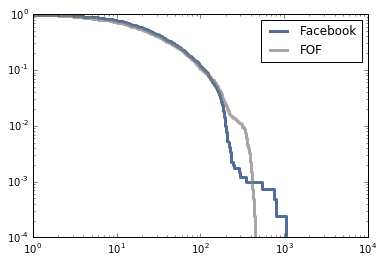And a good match for the rest of the distribution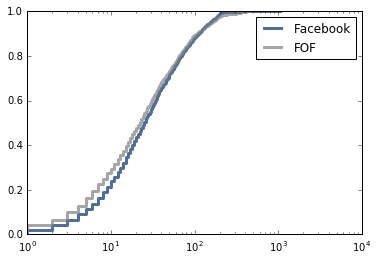In summary, the FOF model has

• Short path lengths, like WS, BA, and HK.
• Moderate clustering, similar to HK, less than WS, and higher than BA.
• Good fit to the tail of the degree distribution, like BA and HK.
• Good fit to the rest of the degree distribution, unlike WS, BA, and HK.

Also, the mechanism of growth is plausible: when a person joins the network, they connect to a randomly-chosen friend and then a random subset of "friends of friends". This process has preferential attachment because friends of friends are more likely to have high degree (see The Inspection Paradox is Everywhere) But the resulting distribution is approximately lognormal, which is heavy tailed, but does not have a power law tail.

### Implementation

Here is a function that generates FOF graphs:

def fof_graph(n, m, p=0.25, seed=None):
if m < 1 or  m+1 >= n:
raise nx.NetworkXError()
if seed is not None:
random.seed(seed)

G = nx.complete_graph(m+1)

for source in range(len(G), n):
# choose a random node
target = random.choice(G.nodes())

# enumerate neighbors of target and add triangles
friends = G.neighbors(target)
k = len(friends)
for friend in friends:
if flip(p):
triangle(G, source, friend)

# connect source and target

return G

def flip(p):
return random.random() < p

def triangle(G, source, friend):
"""Chooses a random neighbor of `friend` and makes a triangle.

Triangle connects `source`, `friend`, and
random neighbor of `friend`.
"""
fof = set(G[friend])
if source in G:
fof -= set(G[source])
if fof:
w = random.choice(list(fof))